# 2D Transformation using CSS

CSS | 2D Transformation: Here, we are going to learn about the 2D (Two-Dimensional) Transformation using CSS (Cascading Style Sheet).
Submitted by Anjali Singh, on December 13, 2019

## CSS | 2D Transformation

2D transformation in CSS allows you to move, rotate, scale, and skew elements shape, size and position along the x-axis and y-axis.

In CSS, 2D transform property allows you to use the following 2D transformation methods,

1. rotate()
2. translate()
3. scale()
4. Skew()
5. matrix()

Let's look at each method one by one.

### 1) rotate()

rotate() property defines a transformation that moves the element around a fixed point on the x-axis in either clockwise or anti-clockwise direction.

Syntax:

```Element{
transform:rotate(30deg);
}
```

Example:

```<!DOCTYPE html>
<html>

<style>
div {
width: 300px;
height: 100px;
background-color: pink;
border: 1px solid black;
}

div#myDiv {
transform: rotate(45deg);
}
</style>

<body>
<div>
this is div
</div>
<div id="myDiv">
this div element is rotated clockwise 45 degrees.
</div>
</body>

</html>
```

Output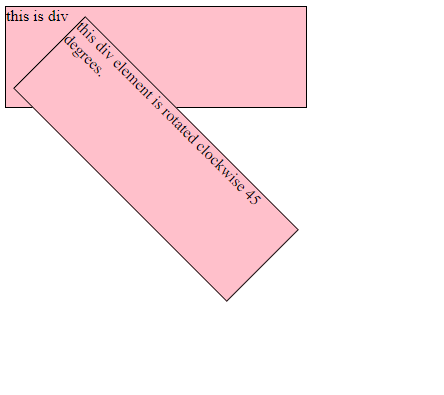In the above example, the <div> element is rotated clockwise 45 degrees.

### 2) translate()

translate() property moves the position of the element from the current position on the X-axis and Y-axis.

• translateX() - Moves the position of the element on the X-axis.
• translateY() - Moves the position of the element on the Y-axis.

Syntax:

```Element{
transform:translate(30px,10px);
}
```

Example:

```<!DOCTYPE html>

<html>
<style>
div {
width: 300px;
height: 100px;
background-color: pink;
border: 1px solid black;
transform: translate(60px, 80px);
}
</style>

<body>
<h1>2D transformation</h1>
<div>
This is a div element.
</div>
</body>

</html>
```

Output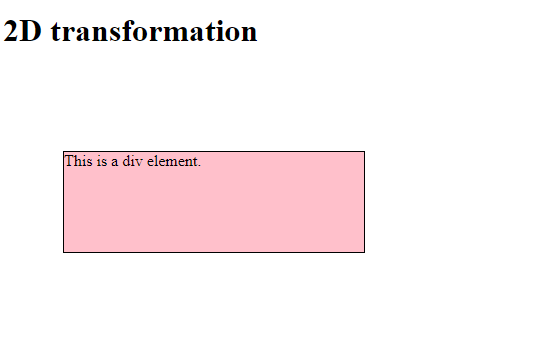In the above example, <div> element 60 pixels to the right, and 80 pixels down from its current position.

### 3) scale()

scale() property modifies the size of the element on the X and Y-axis.

scaleX() - Modifies the size of the element on the X-axis. scaleY() - Modifies the size of the element on the Y-axis.

Syntax:

```Element{
transform:scale(2,3);
}
```

Example:

```<!DOCTYPE html>

<html>

<style>
div {
margin: 150px;
width: 200px;
height: 100px;
background-color: pink;
border: 1px solid black;
transform: scale(2, 3);
}
</style>

<body>
<h1>2D transformation</h1>
<div>
This is div element.
</div>
</body>

</html>
```

Output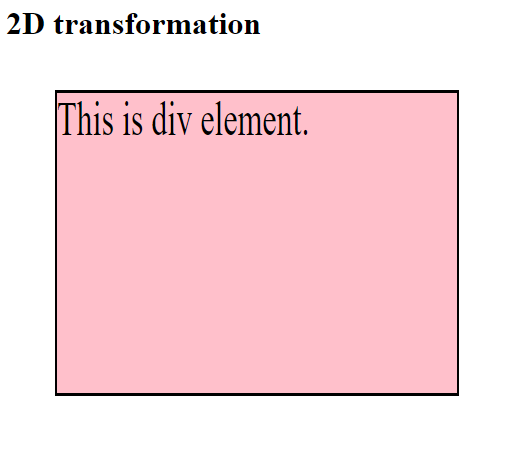In the above example, element <div> increases to 3 times of its original height, and 2 times of its original width.

### 4) skew()

Shear mapping or skewing each point of an element by a certain angle along the X-axis or Y-axis.

• skewX() - Horizontally skewing each point of an element by a certain angle in the horizontal direction.
• skewY() - Vertically skewing each point of an element by a certain angle in the vertical direction.

Syntax:

```Element{
transform:skew(3,4);
}
```

Example:

```<!DOCTYPE html>
<html>

<style>
div {
margin: 150px;
width: 200px;
height: 100px;
background-color: pink;
border: 1px solid black;
transform: scale(1, 2);
}
</style>

<body>
<h1>2D transformation</h1>
<div>
This is div element.
</div>
</body>

</html>
```

Output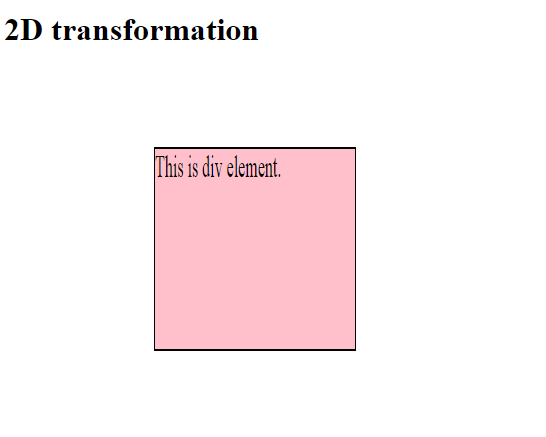In the above example, skews the <div> element 1px along the X-axis, and 2px along the Y-axis.

### 5) matrix()

matrix() property defines a 2D transformation in the form of a transformation matrix. The method matrix() takes six parameters which allow you to rotate, scale, translate, and skew elements in respective order.

Syntax:

```Element{
transform:matrix(1, -0.3, 0, 1, 0, 0);
}
```

Example:

```<!DOCTYPE html>
<html>

<style>
div {
width: 300px;
height: 100px;
background-color: pink;
border: 1px solid black;
transform: matrix(1, -0.3, 0, 1, 0, 0);
}
</style>

<body>
<h1>2D transformation</h1>
<div>
This a div element..
</div>
</body>

</html>
```

Output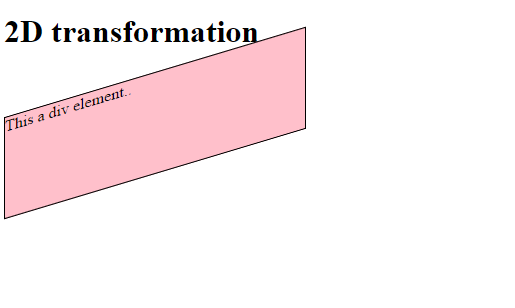In the above example, matrix() property is used.